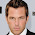## Tuesday, June 8, 2010

### Creating a Custom PatternCreating custom patterns is an easy way to add detail to a project and make it more presentable. Getting the pattern you desire, well that's a whole other story. It is as complicated as it can be. The image above is one of our custom tile patterns. I will explain how we got to this pattern later but before I do so, I think it is more important to decode the process of making the code. The way a pattern is made is through a series of numbers, or as Revit calls it, a pattern descriptor.

The image below is my attempt at understanding what the pattern descriptor does. And as a note, it is slightly different for horizontal lines and vertical lines, I will explain how.The first number as can be seen from the above image, is the angle. As we all know a '0' means it's horizontal and a '90' means it's vertical. And of course any other angle in between can also be used such as '45', '30' and so forth.

The next set of numbers are the origin. Think back to math class, plotting points on a graph. In this example I started at (0, 0,).

The numbers that follow are the x-shift and y-shift numbers. These numbers are what set the starting point. The example above starts at (5, 5). The y-shift number is the desired spacing between each horizontal line. I will discuss how to determine the x-shift number further down.

The pen down number is the length of the line. I set it at 3. So I now have a new point at (8, 5), which creates a line between these two points.

The pen up is the spacing between each line segment. This number is always a negative. A -7 determines a new starting point at (15, 5) and due to the pen down number, an ending point at (18, 5). This sequence keeps going over and over. I will also discuss how this number is determined further down.

The x-shift number aside from determining the starting point on the x-axis also tells the line above to shift over, therefore creating a staggering pattern as it moves upward. A '0' for the x-shift would align the line segments vertically. This number can be any you desired but if a *Gap is desired, this number is also a contributor to creating it as explained below.

* In this example there's a gap between each line segment as shown above. This is determined by the following 2 conditions:

1. X-shift = Pen Dn + Desired SP
2. Pen Up = Pen Dn + Desired SP + Desired SP

The desired spacing (SP) is what you want the gap to be. In this example I want the gap to be 2 inches each way and my pen down to be 3. So once these two numbers are determined I can placed in them in the two formulas above.

X-shift = 3 + 2 = 5

-Pen Up = 3 + 2 + 2 = -7

These are now my x-shift and pen up numbers.

The horizontal lines are drawn once all the data above is inputted.

To draw the vertical lines, the process is exactly the same except for one exception. The x-shift and y-shift numbers are switch. The first number is no longer the x-shift, it's the y-shift. See the image below. I hope this helps and answers the dreaded question of how do I change the pattern. If you have any questions, please ask and I will do my best to answer it. I will post another blog of how we got our pattern shown on the first image.#### 1 comment:

1.Its a nice informative post and its great resource for lots of peoples, so to promote your business by using some internet marketing strategy and it can easily to reach the correct market place.customized pens